# 再也不怕女朋友问我二分查找了！【手绘漫画】图解二分查找（修订版）（LeetCode 704题）# 图解算法与数据结构

## 1、前言## 2、代码

// C
int lower_bound(int* nums, int numsSize, int target){
int left = 0;
int right = numsSize-1;
while(left < right){	// 搜索区间[first, last)不为空
// 防溢出
int mid = left + right >> 1;
if(nums[mid] >= target) right = mid;
else left = mid + 1;
}
return left;			// right也行，因为[left, right)为空的时候它们重合
}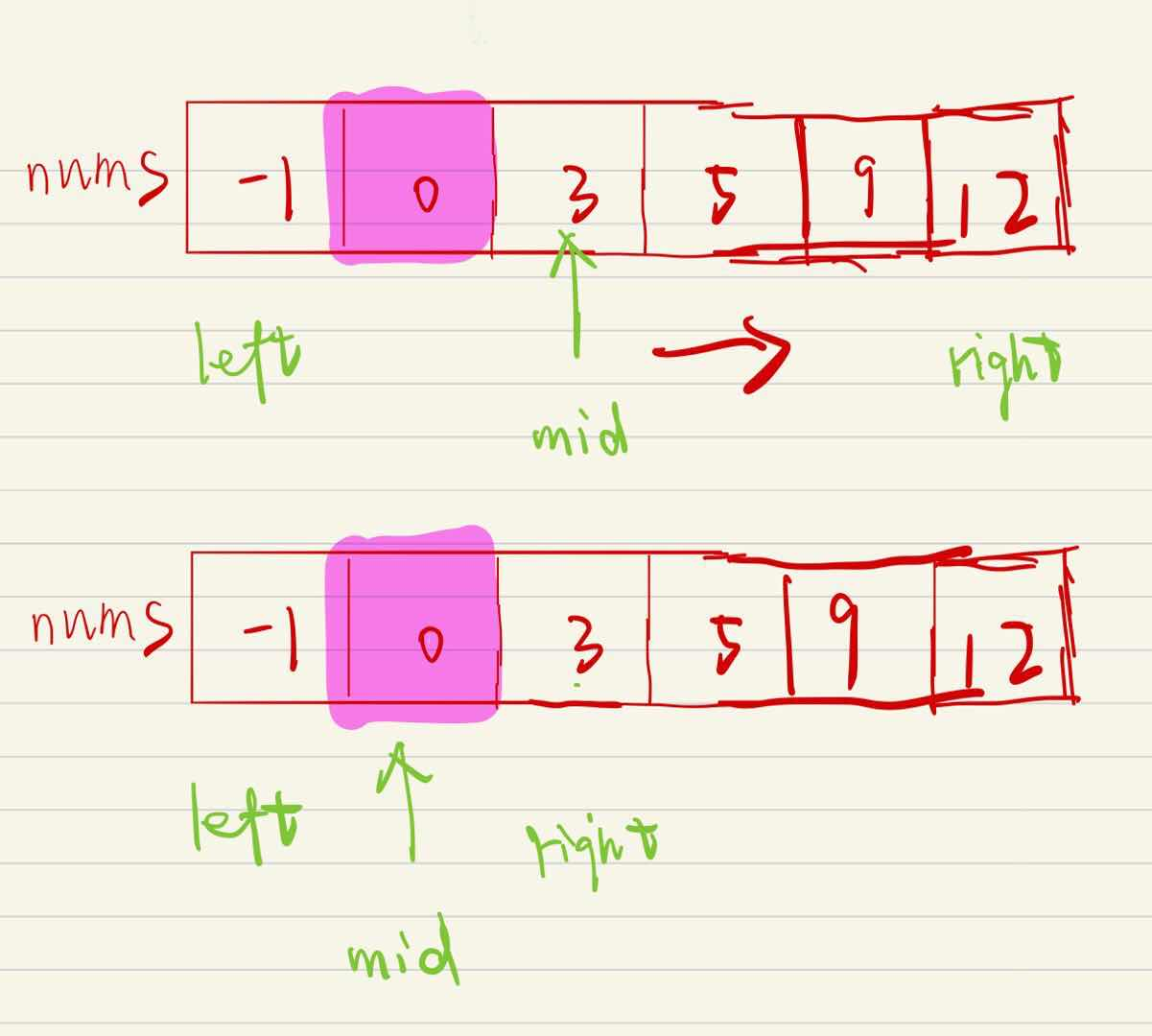// C
int lower_bound(int* nums, int numsSize, int target){
int left = 0;
int right = numsSize-1;
while(left < right){	// 搜索区间[first, last)不为空
// 防溢出
int mid = left + right + 1 >> 1; // 防止死循环，mid加1
if(nums[mid] <= target) left = mid;
else right = mid + 1;
}
return left;			// right也行，因为[left, right)为空的时候它们重合
}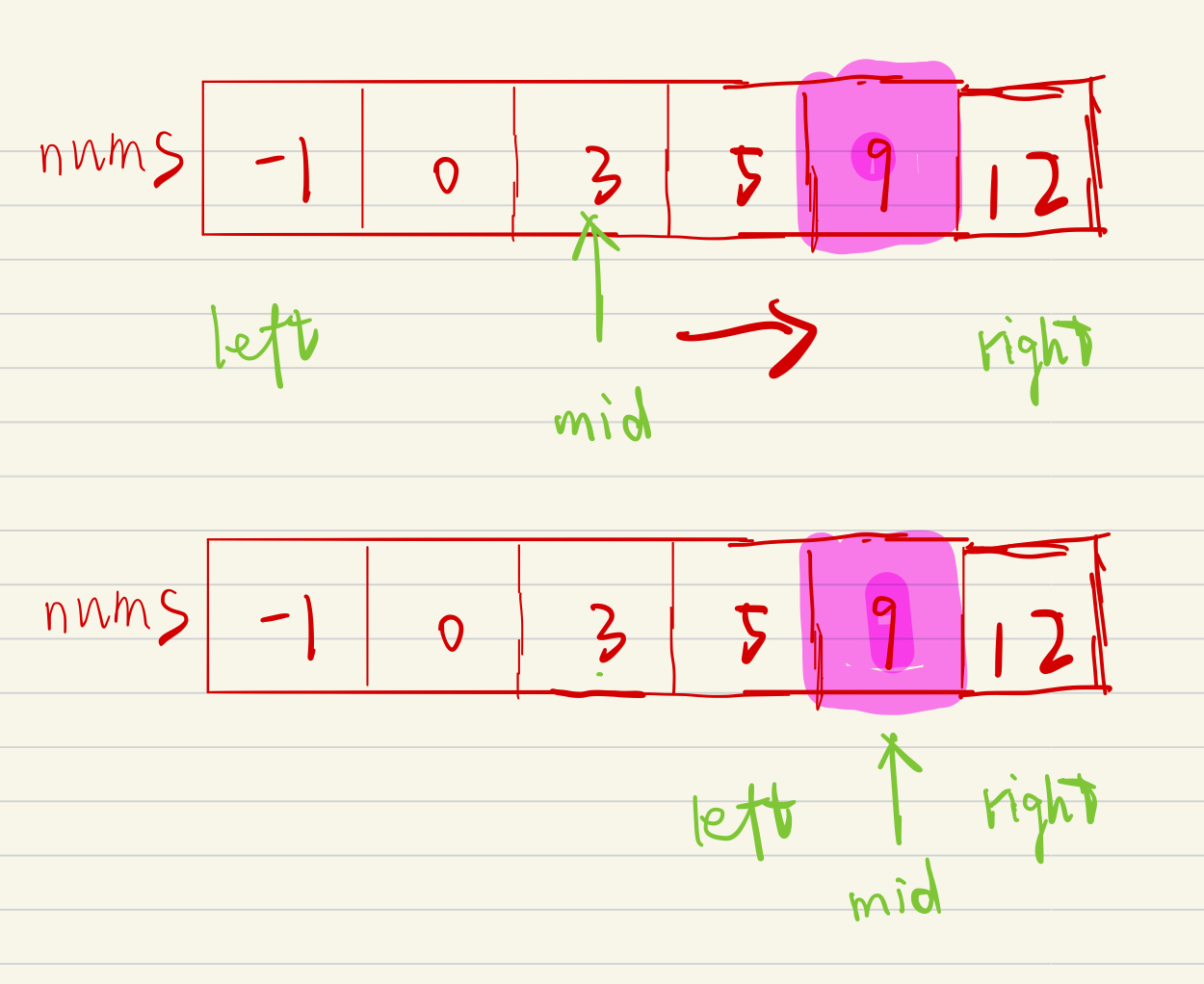• 模板一：区间 [left, right] 划分成 [left, mid][mid + 1, right] 时，其更新操作是 right = mid 或者 left = mid + 1;，计算 mid 时不需要加 1
• 模板二：区间 [left, right] 划分成 [left, mid - 1][mid, right] 时，其更新操作是 r = mid - 1 或者 l = mid;，此时为了防止死循环，计算 mid 时需要加 1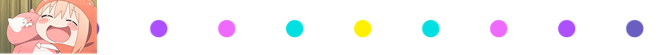## 3、实例（LeetCode 704题）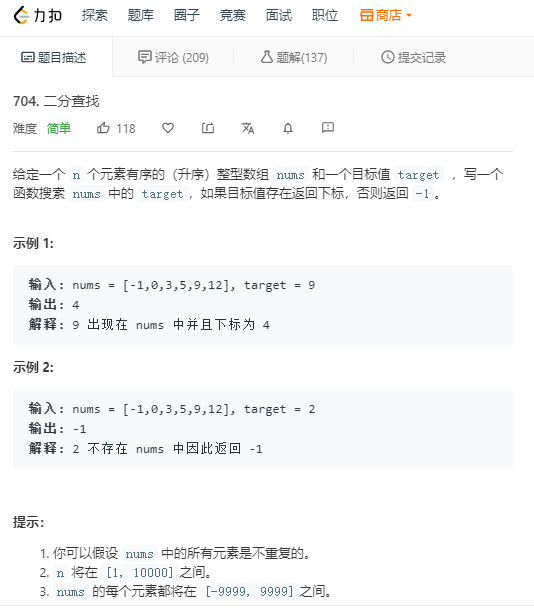int search(int* nums, int numsSize, int target){
int left=0;
int right=numsSize-1;
while(left<right){
int mid=left+right >> 1;
if(nums[mid]>=target){
right=mid;
}
else{
left=mid+1;
}
}
if(nums[left]==target) return left;
return -1;
}// C
int search(int* nums, int numsSize, int target){
int left = 0;
int right = numsSize - 1;

while (left <= right) {
// 最好这么写，不然会内存溢出
// 同时还能减少运行时间！！！
int mid = (left + right) >> 1;
// 等于的情况最简单，首先判断，没准一下就中了！
if (nums[mid] == target) {
return mid;
}
else if (nums[mid] < target) {
// 左边界更新为 mid + 1
left = mid + 1;
}
else {
// 右边界更新为 mid - 1
right = mid - 1;
}
}
return -1;
}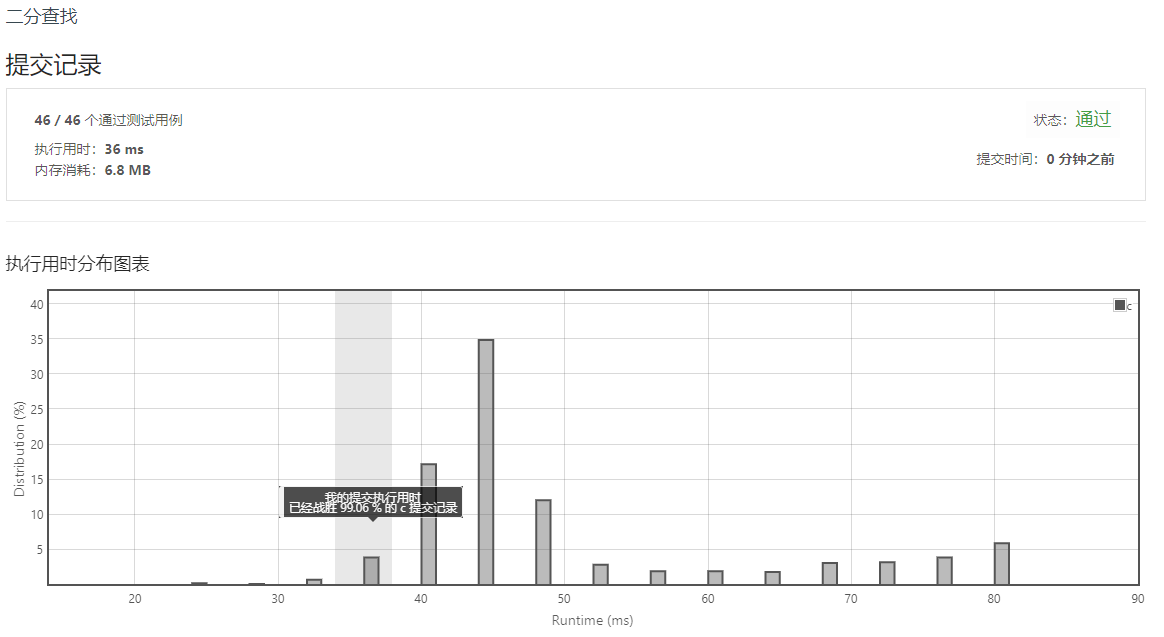03-1020万+04-121443
01-26
02-154万+
09-191万+
04-183688
04-05934
01-1029
09-171万+
07-312272